# Inheritance in C++

• Difficulty Level : Easy
• Last Updated : 23 Jun, 2022

The capability of a class to derive properties and characteristics from another class is called Inheritance. Inheritance is one of the most important features of Object-Oriented Programming.

Inheritance is a feature or a process in which, new classes are created from the existing classes. The new class created is called “derived class” or “child class” and the existing class is known as the “base class” or “parent class”. The derived class now is said to be inherited from the base class.

When we say derived class inherits the base class, it means, the derived class inherits all the properties of the base class, without changing the properties of base class and may add new features to its own. These new features in the derived class will not affect the base class. The derived class is the specialized class for the base class.

• Sub Class: The class that inherits properties from another class is called Subclass or Derived Class.
• Super Class: The class whose properties are inherited by a subclass is called Base Class or Superclass.

The article is divided into the following subtopics:

• Why and when to use inheritance?
• Modes of Inheritance
• Types of Inheritance

### Why and when to use inheritance?

Consider a group of vehicles. You need to create classes for Bus, Car, and Truck. The methods fuelAmount(), capacity(), applyBrakes() will be the same for all three classes. If we create these classes avoiding inheritance then we have to write all of these functions in each of the three classes as shown below figure: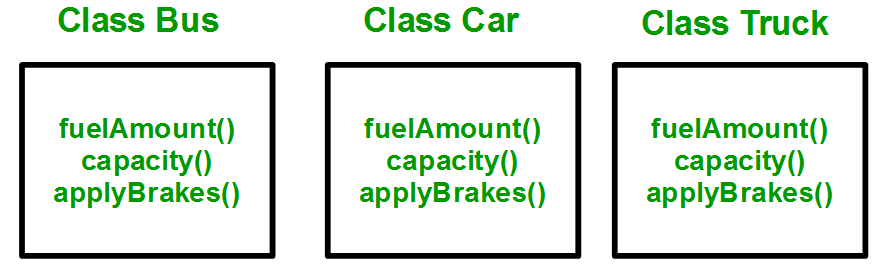You can clearly see that the above process results in duplication of the same code 3 times. This increases the chances of error and data redundancy. To avoid this type of situation, inheritance is used. If we create a class Vehicle and write these three functions in it and inherit the rest of the classes from the vehicle class, then we can simply avoid the duplication of data and increase re-usability. Look at the below diagram in which the three classes are inherited from vehicle class:Using inheritance, we have to write the functions only one time instead of three times as we have inherited the rest of the three classes from the base class (Vehicle).
Implementing inheritance in C++: For creating a sub-class that is inherited from the base class we have to follow the below syntax.

Derived Classes: A Derived class is defined as the class derived from the base class.
Syntax

```class  <derived_class_name> : <access-specifier> <base_class_name>
{
//body
}```

Where
class      — keyword to create a new class
derived_class_name   — name of the new class, which will inherit the base class
access-specifier  — either of private, public or protected. Is neither is specified, PRIVATE is taken as default
base-class-name  — name of the base class
Note: A derived class doesn’t inherit access to private data members. However, it does inherit a full parent object, which contains any private members which that class declares.

Example:
1. class ABC : private XYZ              //private derivation
{                }
2. class ABC : public XYZ              //public derivation
{               }
3. class ABC : protected XYZ              //protected derivation
{              }
4. class ABC: XYZ                            //private derivation by default
{            }

#### Note:

o When a base class is privately inherited by the derived class, public members of the base class becomes the private members of the derived class and therefore, the public members of the base class can only be accessed by the member functions of the derived class. They are inaccessible to the objects of the derived class.
o On the other hand, when the base class is publicly inherited by the derived class, public members of the base class also become the public members of the derived class. Therefore, the public members of the base class are accessible by the objects of the derived class as well as by the member functions of the derived class.

## C++

 `// Example: define member function without argument within the class`   `#include` `using` `namespace` `std;`   `class` `Person` `{` `    ``int` `id;` `    ``char` `name;` `  `  `    ``public``:` `        ``void` `set_p()` `        ``{` `            ``cout<<``"Enter the Id:"``;` `            ``cin>>id;` `            ``fflush``(stdin);` `            ``cout<<``"Enter the Name:"``;` `            ``cin.get(name,100);` `        ``}` `  `  `        ``void` `display_p()` `        ``{` `            ``cout<>fee;` `        ``}` `        `  `        ``void` `display_s()` `        ``{` `            ``display_p();` `            ``cout<<``"t"``<

Output

```Enter the Id:Enter the Name:Enter the Course Name:Enter the Course Fee:
0    t    0```

## C++

 `// Example: define member function without argument outside the class`   `#include` `using` `namespace` `std;`   `class` `Person` `{` `    ``int` `id;` `    ``char` `name;` `  `  `    ``public``:` `        ``void` `set_p();` `        ``void` `display_p();` `};`   `void` `Person::set_p()` `{` `    ``cout<<``"Enter the Id:"``;` `    ``cin>>id;` `    ``fflush``(stdin);` `    ``cout<<``"Enter the Name:"``;` `    ``cin.get(name,100);` `}`   `void` `Person::display_p()` `{` `    ``cout<>fee;` `}`   `void` `Student::display_s()` `{` `    ``display_p();` `    ``cout<<``"t"``<

Output

```Enter the Id:Enter the Name:Enter the Course Name:Enter the Course Fee:
0    t    0```

## C++

 `// Example: define member function with argument outside the class`   `#include` `#include` `using` `namespace` `std;`   `class` `Person` `{` `    ``int` `id;` `    ``char` `name;` `  `  `    ``public``:` `        ``void` `set_p(``int``,``char``[]);` `        ``void` `display_p();` `};`   `void` `Person::set_p(``int` `id,``char` `n[])` `{` `    ``this``->id=id;` `    ``strcpy``(``this``->name,n);        ` `}`   `void` `Person::display_p()` `{` `    ``cout<

## CPP

 `// C++ program to demonstrate implementation` `// of Inheritance`   `#include ` `using` `namespace` `std;`   `// Base class` `class` `Parent {` `public``:` `    ``int` `id_p;` `};`   `// Sub class inheriting from Base Class(Parent)` `class` `Child : ``public` `Parent {` `public``:` `    ``int` `id_c;` `};`   `// main function` `int` `main()` `{` `    ``Child obj1;`   `    ``// An object of class child has all data members` `    ``// and member functions of class parent` `    ``obj1.id_c = 7;` `    ``obj1.id_p = 91;` `    ``cout << ``"Child id is: "` `<< obj1.id_c << ``'\n'``;` `    ``cout << ``"Parent id is: "` `<< obj1.id_p << ``'\n'``;`   `    ``return` `0;` `}`

Output

```Child id is: 7
Parent id is: 91```

Output:

```Child id is: 7
Parent id is: 91```

In the above program, the ‘Child’ class is publicly inherited from the ‘Parent’ class so the public data members of the class ‘Parent’ will also be inherited by the class ‘Child’.
Modes of Inheritance: There are 3 modes of inheritance.

1. Public Mode: If we derive a subclass from a public base class. Then the public member of the base class will become public in the derived class and protected members of the base class will become protected in the derived class.
2. Protected Mode: If we derive a subclass from a Protected base class. Then both public members and protected members of the base class will become protected in the derived class.
3. Private Mode: If we derive a subclass from a Private base class. Then both public members and protected members of the base class will become Private in the derived class.

Note: The private members in the base class cannot be directly accessed in the derived class, while protected members can be directly accessed. For example, Classes B, C, and D all contain the variables x, y, and z in the below example. It is just a question of access.

## CPP

 `// C++ Implementation to show that a derived class` `// doesn’t inherit access to private data members.` `// However, it does inherit a full parent object.` `class` `A {` `public``:` `    ``int` `x;`   `protected``:` `    ``int` `y;`   `private``:` `    ``int` `z;` `};`   `class` `B : ``public` `A {` `    ``// x is public` `    ``// y is protected` `    ``// z is not accessible from B` `};`   `class` `C : ``protected` `A {` `    ``// x is protected` `    ``// y is protected` `    ``// z is not accessible from C` `};`   `class` `D : ``private` `A ``// 'private' is default for classes` `{` `    ``// x is private` `    ``// y is private` `    ``// z is not accessible from D` `};`

The below table summarizes the above three modes and shows the access specifier of the members of the base class in the subclass when derived in public, protected and private modes: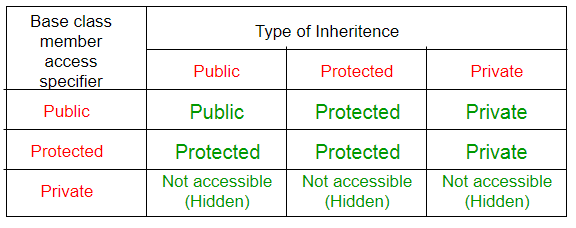### Types Of Inheritance:-

1. Single inheritance
2. Multilevel inheritance
3. Multiple inheritance
4. Hierarchical inheritance
5. Hybrid inheritance

### Types of Inheritance in C++

1. Single Inheritance: In single inheritance, a class is allowed to inherit from only one class. i.e. one subclass is inherited by one base class only.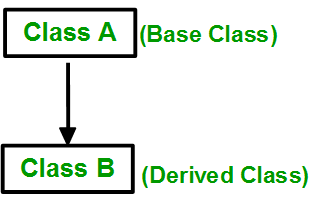Syntax

```class subclass_name : access_mode base_class
{
// body of subclass
};

OR

class A
{
... .. ...
};

class B: public A
{
... .. ...
};```

## CPP

 `// C++ program to explain ` `// Single inheritance` `#include` `using` `namespace` `std;`   `// base class` `class` `Vehicle {` `  ``public``:` `    ``Vehicle()` `    ``{` `      ``cout << ``"This is a Vehicle\n"``;` `    ``}` `};`   `// sub class derived from a single base classes` `class` `Car : ``public` `Vehicle {`   `};`   `// main function` `int` `main()` `{   ` `    ``// Creating object of sub class will` `    ``// invoke the constructor of base classes` `    ``Car obj;` `    ``return` `0;` `}`

Output

`This is a Vehicle`

## C++

 `// Example:`   `#include` `using` `namespace` `std;`   `class` `A` `{` `    ``protected``:` `    ``int` `a;` `  `  `    ``public``:` `        ``void` `set_A()` `        ``{` `            ``cout<<``"Enter the Value of A="``;` `            ``cin>>a;` `            `  `        ``}` `        ``void` `disp_A()` `        ``{` `            ``cout<

Output:- Enter the Value of A= 3 3 Enter the Value of B= 5 5 Product of 3 * 5 = 15

## C++

 `// Example:`   `#include` `using` `namespace` `std;`   `class` `A` `{` `    ``protected``:` `    ``int` `a;` `  `  `    ``public``:` `        ``void` `set_A(``int` `x)` `        ``{` `             ``a=x;            ` `        ``}` `  `  `        ``void` `disp_A()` `        ``{` `            ``cout<

Output

`Product of 4 * 5 = 20`

2. Multiple Inheritance: Multiple Inheritance is a feature of C++ where a class can inherit from more than one class. i.e one subclass is inherited from more than one base class.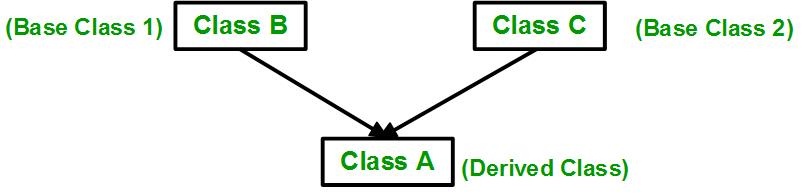Syntax

```class subclass_name : access_mode base_class1, access_mode base_class2, ....
{
// body of subclass
};

class B
{
... .. ...
};
class C
{
... .. ...
};
class A: public B, public C
{
... ... ...
};```

Here, the number of base classes will be separated by a comma (‘, ‘) and the access mode for every base class must be specified.

## CPP

 `// C++ program to explain` `// multiple inheritance` `#include ` `using` `namespace` `std;`   `// first base class` `class` `Vehicle {` `public``:` `    ``Vehicle() { cout << ``"This is a Vehicle\n"``; }` `};`   `// second base class` `class` `FourWheeler {` `public``:` `    ``FourWheeler()` `    ``{` `        ``cout << ``"This is a 4 wheeler Vehicle\n"``;` `    ``}` `};`   `// sub class derived from two base classes` `class` `Car : ``public` `Vehicle, ``public` `FourWheeler {` `};`   `// main function` `int` `main()` `{` `    ``// Creating object of sub class will` `    ``// invoke the constructor of base classes.` `    ``Car obj;` `    ``return` `0;` `}`

Output

```This is a Vehicle
This is a 4 wheeler Vehicle```

## C++

 `// Example:`   `#include` `using` `namespace` `std;`   `class` `A` `{` `              ``protected``:` `              ``int` `a;` `  `  `              ``public``:` `                  ``void` `set_A()` `                  ``{` `                        ``cout<<``"Enter the Value of A="``;` `                        ``cin>>a;` `         `  `                  ``}`   `                  ``void` `disp_A()` `                  ``{` `                        ``cout<

To know more about it, please refer to the article Multiple Inheritances

3. Multilevel Inheritance: In this type of inheritance, a derived class is created from another derived class.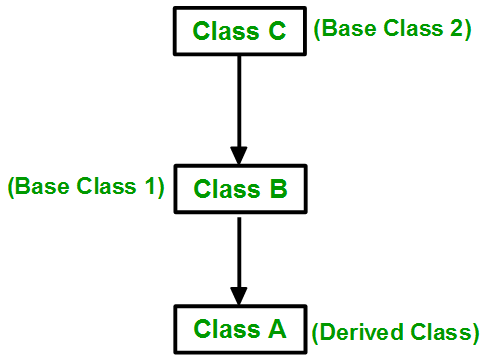Syntax:-

```class C
{
... .. ...
};
class B:public C
{
... .. ...
};
class A: public B
{
... ... ...
};```

## CPP

 `// C++ program to implement` `// Multilevel Inheritance` `#include ` `using` `namespace` `std;`   `// base class` `class` `Vehicle {` `public``:` `    ``Vehicle() { cout << ``"This is a Vehicle\n"``; }` `};`   `// first sub_class derived from class vehicle` `class` `fourWheeler : ``public` `Vehicle {` `public``:` `    ``fourWheeler()` `    ``{` `        ``cout << ``"Objects with 4 wheels are vehicles\n"``;` `    ``}` `};` `// sub class derived from the derived base class fourWheeler` `class` `Car : ``public` `fourWheeler {` `public``:` `    ``Car() { cout << ``"Car has 4 Wheels\n"``; }` `};`   `// main function` `int` `main()` `{` `    ``// Creating object of sub class will` `    ``// invoke the constructor of base classes.` `    ``Car obj;` `    ``return` `0;` `}`

Output

```This is a Vehicle
Objects with 4 wheels are vehicles
Car has 4 Wheels```

4. Hierarchical Inheritance: In this type of inheritance, more than one subclass is inherited from a single base class. i.e. more than one derived class is created from a single base class.Syntax:-

```class A
{
// body of the class A.
}
class B : public A
{
// body of class B.
}
class C : public A
{
// body of class C.
}
class D : public A
{
// body of class D.
}   ```

## CPP

 `// C++ program to implement` `// Hierarchical Inheritance` `#include ` `using` `namespace` `std;`   `// base class` `class` `Vehicle {` `public``:` `    ``Vehicle() { cout << ``"This is a Vehicle\n"``; }` `};`   `// first sub class` `class` `Car : ``public` `Vehicle {` `};`   `// second sub class` `class` `Bus : ``public` `Vehicle {` `};`   `// main function` `int` `main()` `{` `    ``// Creating object of sub class will` `    ``// invoke the constructor of base class.` `    ``Car obj1;` `    ``Bus obj2;` `    ``return` `0;` `}`

Output

```This is a Vehicle
This is a Vehicle```

5. Hybrid (Virtual) Inheritance: Hybrid Inheritance is implemented by combining more than one type of inheritance. For example: Combining Hierarchical inheritance and Multiple Inheritance.
Below image shows the combination of hierarchical and multiple inheritances: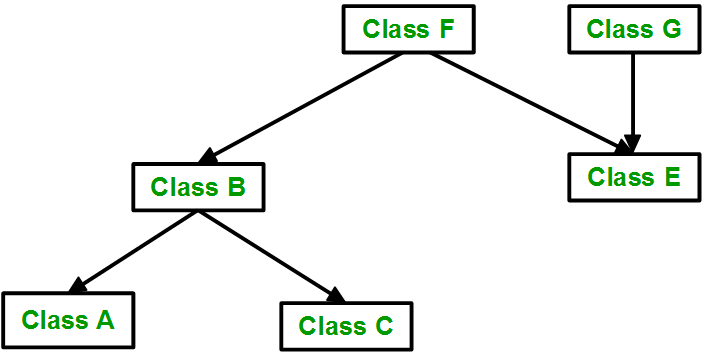## CPP

 `// C++ program for Hybrid Inheritance`   `#include ` `using` `namespace` `std;`   `// base class` `class` `Vehicle {` `public``:` `    ``Vehicle() { cout << ``"This is a Vehicle\n"``; }` `};`   `// base class` `class` `Fare {` `public``:` `    ``Fare() { cout << ``"Fare of Vehicle\n"``; }` `};`   `// first sub class` `class` `Car : ``public` `Vehicle {` `};`   `// second sub class` `class` `Bus : ``public` `Vehicle, ``public` `Fare {` `};`   `// main function` `int` `main()` `{` `    ``// Creating object of sub class will` `    ``// invoke the constructor of base class.` `    ``Bus obj2;` `    ``return` `0;` `}`

Output

```This is a Vehicle
Fare of Vehicle```

## C++

 `// Example:`   `#include   ` `using` `namespace` `std;  `   `class` `A  ` `{  ` `    ``protected``:  ` `    ``int` `a;  ` `    ``public``:  ` `    ``void` `get_a()  ` `    ``{  ` `       ``cout << ``"Enter the value of 'a' : "``;  ` `       ``cin>>a;  ` `    ``}  ` `};  ` `  `  `class` `B : ``public` `A   ` `{  ` `    ``protected``:  ` `    ``int` `b;  ` `    ``public``:  ` `    ``void` `get_b()  ` `    ``{  ` `       ``cout << ``"Enter the value of 'b' : "``;` `       ``cin>>b;  ` `    ``}  ` `};  ` `class` `C   ` `{  ` `    ``protected``:  ` `    ``int` `c;  ` `    ``public``:  ` `    ``void` `get_c()  ` `    ``{  ` `        ``cout << ``"Enter the value of c is : "``;  ` `        ``cin>>c;  ` `    ``}  ` `};  ` `  `  `class` `D : ``public` `B, ``public` `C  ` `{  ` `    ``protected``:  ` `    ``int` `d;  ` `    ``public``:  ` `    ``void` `mul()  ` `    ``{  ` `         ``get_a();  ` `         ``get_b();  ` `         ``get_c();  ` `         ``cout << ``"Multiplication of a,b,c is : "` `<

6. A special case of hybrid inheritance: Multipath inheritance
A derived class with two base classes and these two base classes have one common base class is called multipath inheritance. Ambiguity can arise in this type of inheritance.
Example:

## CPP

 `// C++ program demonstrating ambiguity in Multipath` `// Inheritance`   `#include ` `using` `namespace` `std;`   `class` `ClassA {` `public``:` `    ``int` `a;` `};`   `class` `ClassB : ``public` `ClassA {` `public``:` `    ``int` `b;` `};`   `class` `ClassC : ``public` `ClassA {` `public``:` `    ``int` `c;` `};`   `class` `ClassD : ``public` `ClassB, ``public` `ClassC {` `public``:` `    ``int` `d;` `};`   `int` `main()` `{` `    ``ClassD obj;`   `    ``// obj.a = 10;                  // Statement 1, Error` `    ``// obj.a = 100;                 // Statement 2, Error`   `    ``obj.ClassB::a = 10; ``// Statement 3` `    ``obj.ClassC::a = 100; ``// Statement 4`   `    ``obj.b = 20;` `    ``obj.c = 30;` `    ``obj.d = 40;`   `    ``cout << ``" a from ClassB  : "` `<< obj.ClassB::a;` `    ``cout << ``"\n a from ClassC  : "` `<< obj.ClassC::a;`   `    ``cout << ``"\n b : "` `<< obj.b;` `    ``cout << ``"\n c : "` `<< obj.c;` `    ``cout << ``"\n d : "` `<< obj.d << ``'\n'``;` `}`

Output

``` a from ClassB  : 10
a from ClassC  : 100
b : 20
c : 30
d : 40```

Output:

```a from ClassB : 10
a from ClassC : 100
b : 20
c : 30
d : 40```

In the above example, both ClassB and ClassC inherit ClassA, they both have a single copy of ClassA. However Class-D inherits both ClassB and ClassC, therefore Class-D has two copies of ClassA, one from ClassB and another from ClassC.
If we need to access the data member of ClassA through the object of Class-D, we must specify the path from which a will be accessed, whether it is from ClassB or ClassC, bcoz compiler can’t differentiate between two copies of ClassA in Class-D.

### There are 2 Ways to Avoid this Ambiguity:

1) Avoiding ambiguity using the scope resolution operator: Using the scope resolution operator we can manually specify the path from which data member a will be accessed, as shown in statements 3 and 4, in the above example.

## CPP

 `obj.ClassB::a = 10;       ``// Statement 3` `obj.ClassC::a = 100;      ``// Statement 4`

Note: Still, there are two copies of ClassA in Class-D.
2) Avoiding ambiguity using the virtual base class:

## CPP

 `#include`   `class` `ClassA` `{` `  ``public``:` `    ``int` `a;` `};`   `class` `ClassB : ``virtual` `public` `ClassA` `{` `  ``public``:` `    ``int` `b;` `};`   `class` `ClassC : ``virtual` `public` `ClassA` `{` `  ``public``:` `    ``int` `c;` `};`   `class` `ClassD : ``public` `ClassB, ``public` `ClassC` `{` `  ``public``:` `    ``int` `d;` `};`   `int` `main()` `{` `    ``ClassD obj;`   `    ``obj.a = 10;       ``// Statement 3` `    ``obj.a = 100;      ``// Statement 4`   `    ``obj.b = 20;` `    ``obj.c = 30;` `    ``obj.d = 40;`   `    ``cout << ``"\n a : "` `<< obj.a;` `    ``cout << ``"\n b : "` `<< obj.b;` `    ``cout << ``"\n c : "` `<< obj.c;` `    ``cout << ``"\n d : "` `<< obj.d << ``'\n'``;` `}`

Output:

```a : 100
b : 20
c : 30
d : 40```

According to the above example, Class-D has only one copy of ClassA, therefore, statement 4 will overwrite the value of a, given in statement 3.

This article is contributed by Harsh Agarwal. If you like GeeksforGeeks and would like to contribute, you can also write an article using write.geeksforgeeks.org or mail your article to contribute@geeksforgeeks.org. See your article appearing on the GeeksforGeeks main page and help other Geeks.

My Personal Notes arrow_drop_up
Recommended Articles
Page :